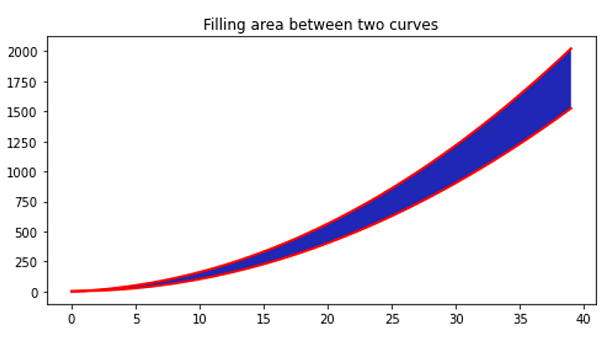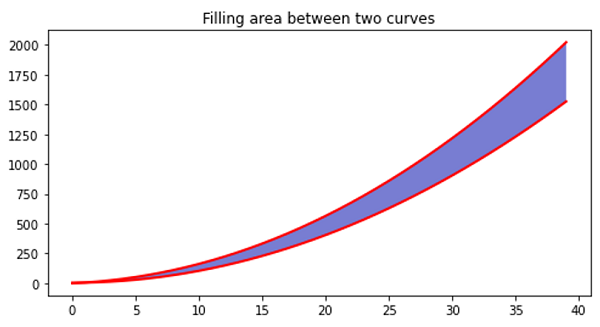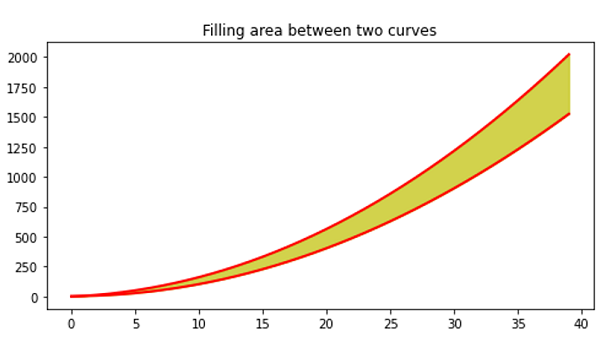# Python | Filling the area between two curves

In this article, we are going to learn how to fill the area between two curves in x-y plot using matplotlib in Python?
Submitted by Anuj Singh, on August 28, 2020

Illustrations:## Python code for filling the area between two curves

```import numpy as np
import matplotlib.pyplot as plt

# Definiing Curves
x = np.arange(40)
y = 4 + x**2
yy = x**2.05 + 5*x

# Filling Area
plt.figure(figsize=(8,4))
plt.fill_between(x, y, yy)
plt.plot(x,y, linewidth=2.0, color='red')
plt.plot(x,yy, linewidth=2.0, color='red')
plt.title('Filling area between two curves')

# Filling Area
plt.figure(figsize=(8,4))
plt.fill_between(x, y, yy, alpha=0.6)
plt.plot(x,y, linewidth=2.0, color='red')
plt.plot(x,yy, linewidth=2.0, color='red')
plt.title('Filling area between two curves')

# Filling Area
plt.figure(figsize=(8,4))
plt.fill_between(x, y, yy, color='y', alpha=0.7)
plt.plot(x,y, linewidth=2.0, color='red')
plt.plot(x,yy, linewidth=2.0, color='red')
plt.title('Filling area between two curves')
```

Output:

```Output is as Figure
```